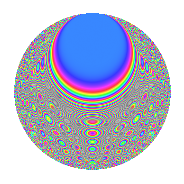# Properties

 Label 1050.2.bfLevel 1050 Weight 2 Character orbit bf Rep. character $$\chi_{1050}(107,\cdot)$$ Character field $$\Q(\zeta_{12})$$ Dimension 192 Sturm bound 480

# Related objects

## Defining parameters

 Level: $$N$$ = $$1050 = 2 \cdot 3 \cdot 5^{2} \cdot 7$$ Weight: $$k$$ = $$2$$ Character orbit: $$[\chi]$$ = 1050.bf (of order $$12$$ and degree $$4$$) Character conductor: $$\operatorname{cond}(\chi)$$ = $$105$$ Character field: $$\Q(\zeta_{12})$$ Sturm bound: $$480$$

## Dimensions

The following table gives the dimensions of various subspaces of $$M_{2}(1050, [\chi])$$.

Total New Old
Modular forms 1056 192 864
Cusp forms 864 192 672
Eisenstein series 192 0 192

## Trace form

 $$192q - 16q^{6} - 4q^{7} + O(q^{10})$$ $$192q - 16q^{6} - 4q^{7} + 96q^{16} + 8q^{18} - 24q^{21} + 24q^{22} + 72q^{27} - 4q^{28} + 16q^{31} + 20q^{33} + 48q^{36} + 8q^{37} + 40q^{42} + 64q^{43} - 48q^{46} - 24q^{57} + 28q^{58} - 8q^{61} + 88q^{63} + 16q^{67} - 8q^{72} + 56q^{73} - 16q^{76} + 16q^{78} - 8q^{81} - 16q^{82} - 76q^{87} - 12q^{88} - 24q^{91} - 84q^{93} - 8q^{96} - 104q^{97} + O(q^{100})$$

## Decomposition of $$S_{2}^{\mathrm{new}}(1050, [\chi])$$ into newform subspaces

The newforms in this space have not yet been added to the LMFDB.

## Decomposition of $$S_{2}^{\mathrm{old}}(1050, [\chi])$$ into lower level spaces

$$S_{2}^{\mathrm{old}}(1050, [\chi]) \cong$$ $$S_{2}^{\mathrm{new}}(105, [\chi])$$$$^{\oplus 4}$$$$\oplus$$$$S_{2}^{\mathrm{new}}(210, [\chi])$$$$^{\oplus 2}$$$$\oplus$$$$S_{2}^{\mathrm{new}}(525, [\chi])$$$$^{\oplus 2}$$

## Hecke Characteristic Polynomials

There are no characteristic polynomials of Hecke operators in the database